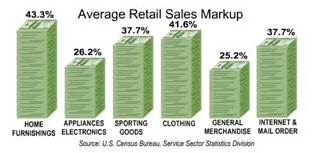# Mark Up versus Margin

Markup vs. Margin

What is the Difference? Is there a difference?

Absolutely!

These two terms are often used interchangeably by folks that do not value accounting skills. However, that misunderstanding can menace of the bottom line of your enterprise. A solid understanding and application of these two separate concepts can enhance our pricing model, and have a drastic effect on the bottom line.

By definition:
• markup percentage is the percentage difference between the actual cost and the selling price.• gross margin percentage is the percentage difference between the selling price and the profit.Photo credit:  Simon Cunningham

Gross margin or gross profit is defined as sales minus cost of goods sold. If a retailer sells a product for \$10 which had a cost of \$8, the gross profit or gross margin is \$2. In our example the gross margin ratio is 20% (\$2 divided by \$10).

In our example, the product had a cost of \$8 and it had a markup of \$2 resulting in a selling price of \$10. The \$2 markup is the same as the \$2 gross profit. However, the markup percentage is often expressed as a percentage of cost. In our example the \$2 markup is divided by the cost of \$8 resulting in a markup of 25%.

Many people have a problem with accountants’ jargon and often get confused between the terms “profit margin” and “markup” which are often bandied about freely or used interchangeably. Although these two terms are used to express different things, they are also, in fact, two different ways of analyzing the cost and profit of a product or service in your small business.

“Profit” is the difference between what you sell it for and what you paid for it. “Margin” simple means you turn that into a percentage of the selling price. You do this so you can compare different items easily.

So the difference is that markup is your profit as a percentage of the cost price and profit margin is your profit as a percentage of your selling price.

For additional information and insight into the fascinating and powerful realm of prices, check these two research-based resources: